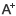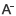# 北师大版一年级数学下册试题日期：2020-01-18     来源：www.zhixueshuo.com    作者：智学网    浏览：519    评论：0

学数学不光动脑，还要勤动手，多做一些训练题会有所提高。下面是学习啦我们收拾的北师大版一年级数学下册试题以供大家阅读。

北师大版一年级数学下册试题

一、 填空题

1. 看图写数.个十和个一，写作

2. 68里面的6在位上，表示个;8在位上，表示个.

3.个十和个一，写作

4. 在○里填上、、=.

17○71　　20○19　　　　 64○46 39○41

5.①个一是十. ②100里面有个十.

③个位是5，十位上是2，这个数是. ④84里面有个十和个一.

6. 在○里填上、或=.

98○89　　　　　90○19　　　 43○34 89○90

7. 在○里填上、、=.

40+7○55-5　　　69-9○40+20 100-60○48-8　　　　　 30+3○20+20

8. 按数的顺序填空.9.和80相邻的数是和.

一位数中最大的是;两位数中最小的是，最大的是.

100是位数.

10. 按数的顺序填空.

41、43、、、

51、53、、、、、、

11. 用5、60、65写出两个加法算式和两个减法算式.

___________________ ____________________

___________________ ____________________

12. 写数，并从小到大排列.

六十七　　　　　 一百　　　　　　　 七十六　　　 五十

十五　　　　　　　　 三十四　　　　 四十三　　　 十一

排列：_____________________________________________

13. 读出下面各数，并按需要填入方格里.

4　　　　1　　　8　　　 12　　　38　　　 100　　　　90　　　 11　　 9

18　　　 20　　 5　　　 10　　　19　　　 91　　　　 49　　　 32　　　 7二、 口算题

1. 　8+70= 2. 　39-4= 3.　 86-80= 4.　 40+5=

87-7= 74-40= 50+3= 6+20=

35-30= 1+60= 22-2= 98-8=

66-6= 32+3= 78-50= 98-80=

三、 应用题

1. 一本书姐姐已经看了50页，还有20页没看，这本书有多少页?

2. 动物园有27只猴子，里面有7只大猴，剩下的是小猴，小猴有多小只?

北师大版一年级数学下册试题

一、口算。

13-9= 　　16-7=　　 12-3=　　 11-8= 　　17-9=　　 18-9= 　　15-8=

16-9= 　　14-5=　　 15-6=　　 11-7= 　　14-7= 　　12-5=　　 13-4=

11-2= 　　12-6= 　　11-9=　　 13-8=　　 11-3= 　　14-9= 　　15-7=

11-4= 　　15-5= 　　5+6=　　 4+9= 　　3+8= 　　11-5=　　 11-6=

12-4=　　 12-7= 　　12-8=　　 12-9= 　　13-5= 　　13-6= 　　13-7=

14-6=　　 14-8= 　　15-9= 　　16-8=　　 17-8= 　　4+7= 　　8+5=

14-9-2=　　 12-7+6= 　　7+6-8= 　　6+3+4= 　　11-9+8= 　　8+8-7=

二、在内填上或=。

15-810 　　11-311-4　　 18-912-2

13-95 　　15-1014-9 　　13-713-6

三、在里填上适合的数。

12-=6 　　4+=13　　 15-=8

7+=10　　 -6=12 　　11-=7

12+=13　　 14-=8　　 5+4=- 6

12-4=-=-=-=-

13-7=-=-=-=-

四、连一连。

8+7　　　10 　　13-10

14-4　　15 　　9-7

6+7　　　3 　　　9+6

7-4　　　13 　　5+8

10-8　　2 　　　5+5

北师大版一年级数学下册试题

一、 填空题。

1. 15-9= 想： 9加等于15， 15减9等于。

2. 12-8= 想： 8加等于12， 12减8等于 。

3.8+=12 　6+=14 　8+=15 　9+=16

二、 口算题。

11-9=　　15-7 =　　12-8 =　　14-6=　　18-9=　　14-10=

11-6=　　13-8=　　15-9=　　18-7=　　16-9=　　12-9=

14-7=　　18-8=　　13-9=　　14-9=　　17-7=　　17-8=

三、算一算，比一比，在○里填上、 或 =。

11-8○4　　 13-8○6-2 　　15- 7○9+1

14-9○5　　 12-7○4+2 　　5+3○13-7

16-7○5+4　　 12-9○4-2　　 5+ 9○18-6

四、算一算，找规律。

我发现

五、看谁写得多。

________________________________________=10

________________________________________=9

________________________________________=6

________________________________________=8

________________________________________=7

0相关评论